## Learning Iterative Reasoning through Energy Minimization

### ICML 2022

Reasoning as Energy Minimization: We formulate reasoning as an optimization process on a learned energy landscape. Above, we illustrate intermediate optimized solutions as we compute the shortest path in a densely connected graph, compute the summation of two 20x20 input matrices, and denoise a noisy input image.

Deep learning has excelled on complex pattern recognition tasks such as image classification and object recognition. However, it struggles with tasks requiring nontrivial reasoning, such as algorithmic computation. Humans are able to solve such tasks through iterative reasoning -- spending more time to think about harder tasks. Most existing neural networks, however, exhibit a fixed computational budget controlled by the neural network architecture, preventing additional computational processing on harder tasks. In this work, we present a new framework for iterative reasoning with neural networks. We train a neural network to parameterize an energy landscape over all outputs, and implement each step of the iterative reasoning as an energy minimization step to find a minimal energy solution. By formulating reasoning as an energy minimization problem, for harder problems that lead to more complex energy landscapes, we may then adjust our underlying computational budget by running a more complex optimization procedure. We empirically illustrate that our iterative reasoning approach can solve more accurate and generalizable algorithmic reasoning tasks in both graph and continuous domains. Finally, we illustrate that our approach can recursively solve algorithmic problems requiring nested reasoning.

## Reasoning through Energy Minimization

Existing approaches torwards iterative reasoning typically rely on the iterative application of a neural network module. In this work, we propose an alternative iterative reasoning approach, Iterative Reasoning as Energy Minimization (IREM), where iterative reasoning is implemented as a iterative optimization process on a learned neural energy landscape parameterized using an EBM. Below, we illustrate this conversion, where the algorithmic operation of addition is converted to an optimization process on a learned energy landscape. The solution of the algorithmic task corresponds to the minimum of the energy landscape. By formulating iterative reasoning in this manner, we obtain a natural termination criteria for reasoning (corresponding to a minimum the energy function). Furthermore, we illustrate how this enables us to adaptively reasoning dependent on the inherent difficulty of the task, and generalize to more complex variants of the task.

## Graphical Reasoning

First, we illustrate how IREM enables iterative reasoning on graphs. We consider graphical tasks such as shortest path and connected components computation. On the bottom left, we illustrate the computation of shortest path using IREM. As sequential optimization steps are run, IREM is able to gradually recover the ground truth shortest path between start node (pictured in green) to a goal node (pictured in blue). On the bottom right, we find that IREM is able to generalize discrete algorithmic computations to significantly larger graphs at test time (performance on up size 30 graphs is shown), when only trained on up to size 10 graphs. IREM exhibits superior performance by utilizing more underlying algorithmic computation steps for more complex problems. In constrast, a recurrent approach is unable to extend algorithmic computation to large graphs, with increased iterative computation failing to improve final performance.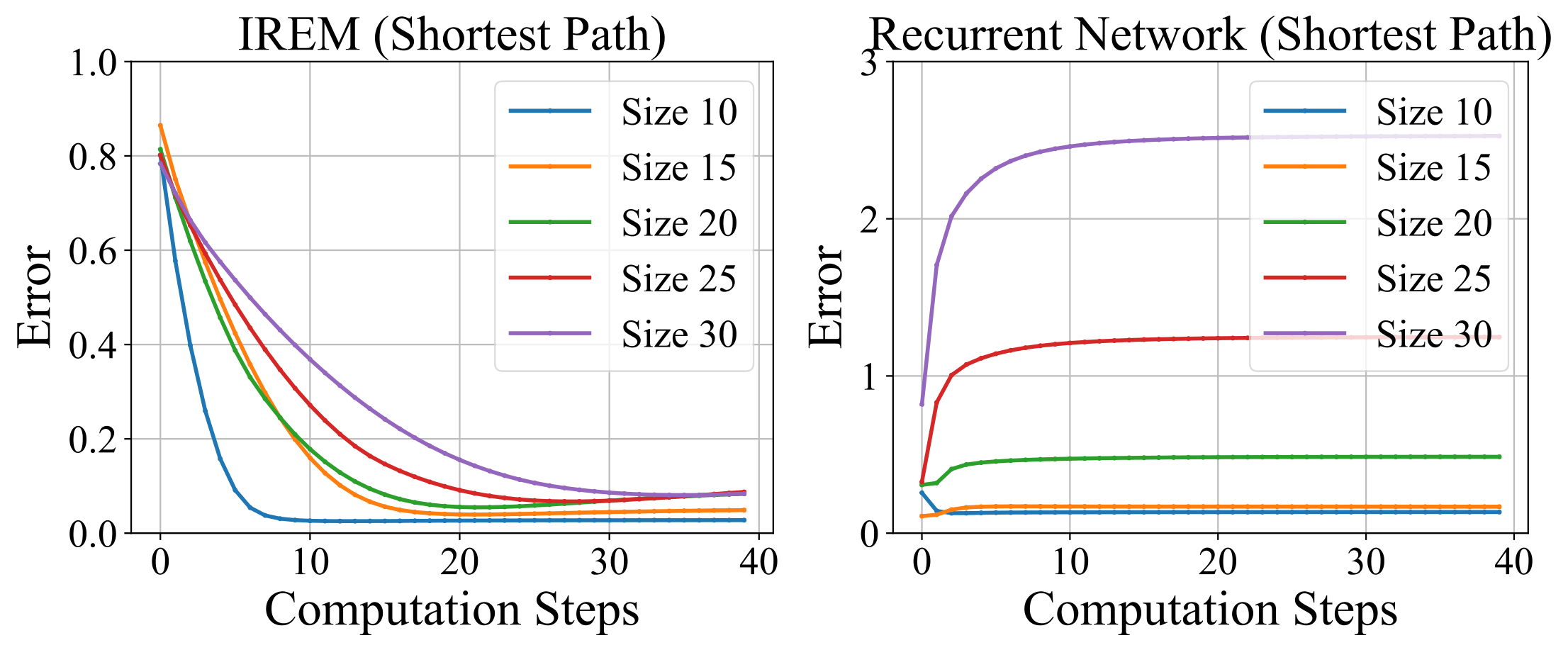## Matrix Reasoning

Next, we further illustrate how IREM enables us to solve algorithmic reasoning tasks on matrix inputs. In the figure below, we illustrate the optimization process on the task of matrix addition, where we seek to add all elements in a 20x20 matrix together. We plot the predicted matrix sum over the course of optimization, as well as the associated error map. Over sequential reasoning steps, we find that IREM is able to compute addition across elements in the matrix nearly perfectly. We find that IREM significantly outperforms baselines across tasks such as matrix addition, matrix inverse, and low-rank matrix completion. We further find that IREM is able to generalize better to harder problem instances than baselines.

Matrix Addition: Illustration of predicted solutions from IREM when adding Matrix A and B, both of size 20x20. As optimization is run, predictions (third column) converge to the correct answer, and the error map (fourth column), consisting of the L1 error of the predicted answer and ground truth answer, decreases to 0.

## Recursive Reasoning

Finally, we illustrate how IREM enables us to recursively apply learned algorithmic computations on multiple inputs. On the right, we consider the addition task, and recursively apply a learned addition operation on up to 10 different 20x20 matrices. As each input is recursively added, the underlying error of intermediate computations increase, and to perform well on this task, models must be able to generalize well to out-of-distribution intermediate computations. Compared to baselines, IREM generalizes substantially better to recursive algorithmic application, exhibiting low computation error and sensible solutions even when up to 10 different matrices are added (see example below on 4 input matrices).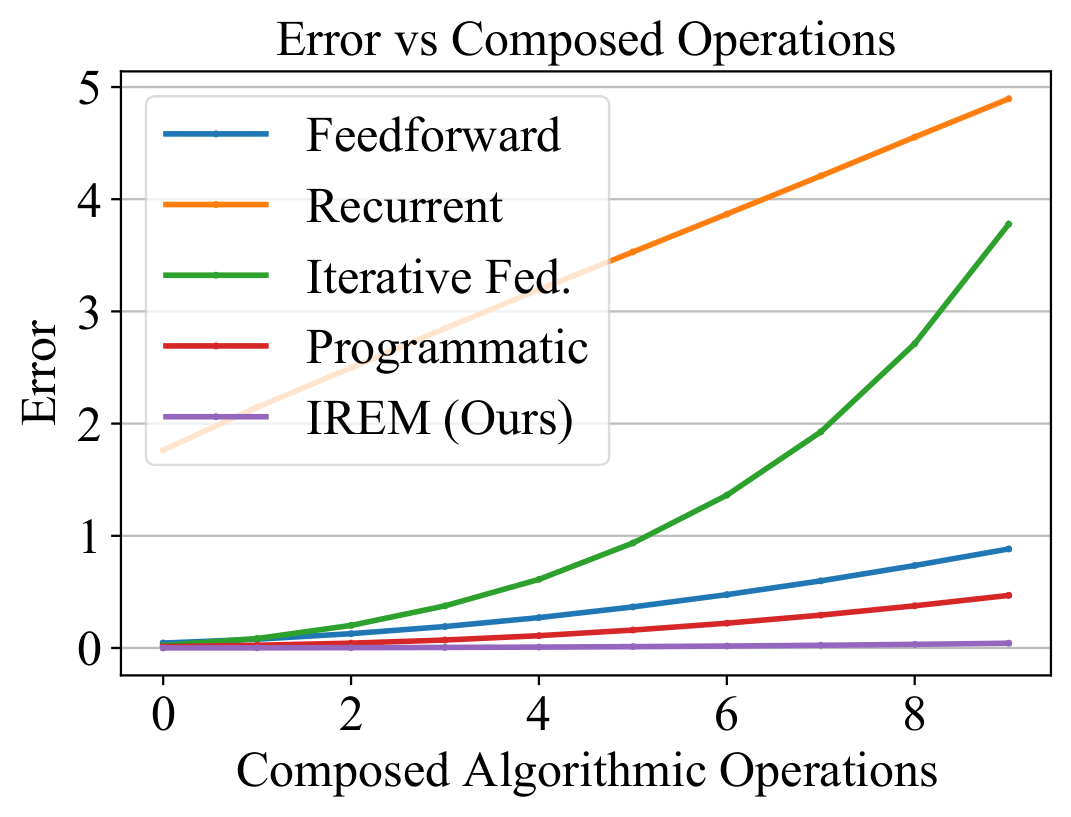Qualitative Composition: Illustration of predicted solutions from IREM when recursively adding four 20x20 matrices. First 9 entries of the predicted 20x20 matrix are shown. Predictions are close to ground truth answer.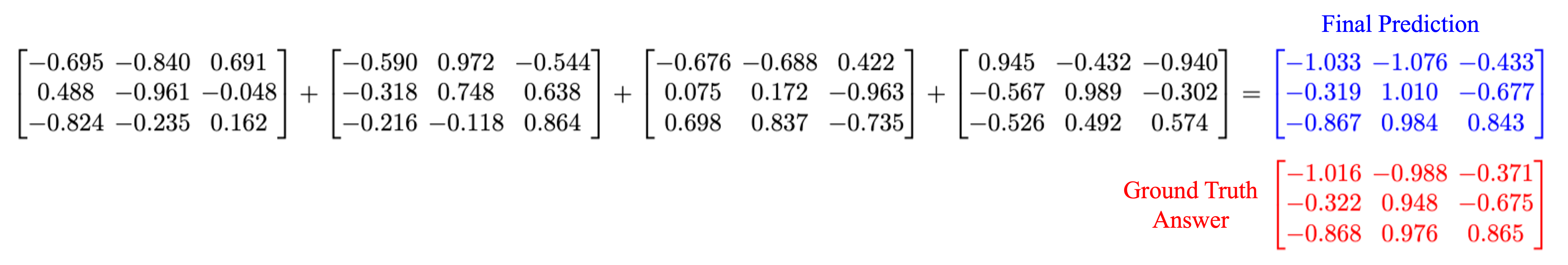## Related Projects

Check out our related projects on energy based models! A full list can be found here.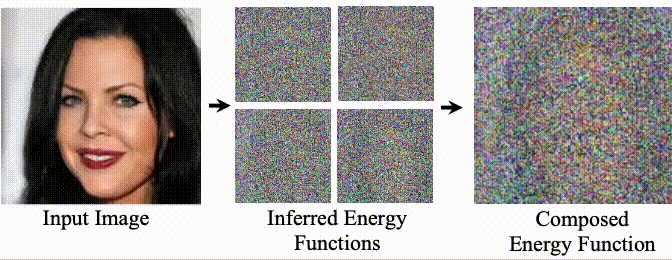We present a framework to decompose images into energy functions representing both global factors of variation as well as local factors of variation. We show such decomposed components generalize well, and are amenable to compositions across different modes of data, and can be further composed with separate energy functions discovered on other datasets.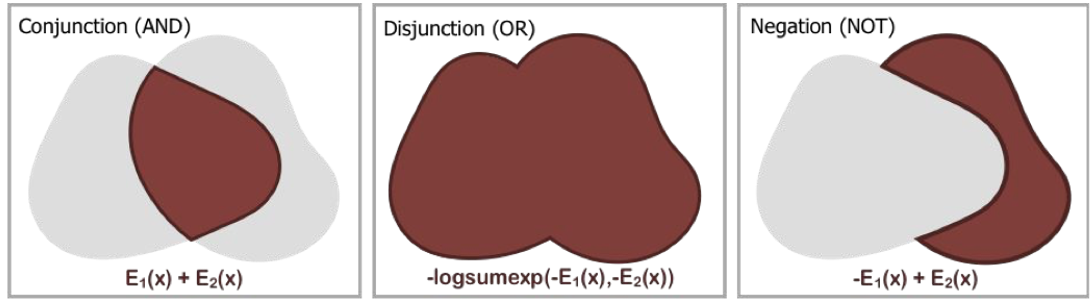We show how EBMs enable zero-shot compositional visual generation, enabling us to compose visual concepts (through operators of conjunction, disjunction, or negation) together in a zero-shot manner. Our approach enables us to generate faces given a description ((Smiling AND Female) OR (NOT Smiling AND Male)) or to combine several different objects together.
We introduce a method to scale EBM training to modern neural network architectures. We show that such trained EBMs have a set of unique properties, enabling model robustness, image and trajectory modeling, continual learning and compositional visual generation.

## Bibtex

@inproceedings{du2022irem, title={Learning Iterative Reasoning through Energy Minimization}, author={Du, Yilun and Li, Shuang and Tenenbaum, B. Joshua and Mordatch, Igor}, booktitle={Proceedings of the 39th International Conference on Machine Learning (ICML-22)}, year={2022} }

Send feedback and questions to Yilun Du